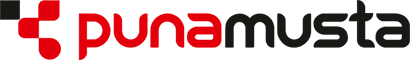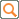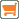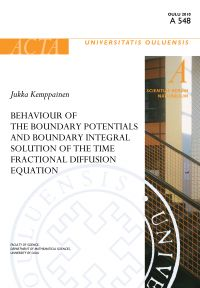Hae:0 tuotetta ostoskorissa

## Oulun yliopiston väitöskirjat# BEHAVIOUR OF THE BOUNDARY POTENTIALS AND BOUNDARY INTEGRAL SOLUTION OF THE TIME FRACTIONAL DIFFUSION EQUATION, ACTA UNIVERSITATIS OULUENSIS A Scientiae Rerum Naturalium 548

 ISBN-13: 978-951-42-6131-2 Kieli: englanti Kustantaja: Oulun yliopisto Oppiaine: Tekniikka, matematiikka Painosvuosi: 2010 Sidosasu: pehmeäkantinen Sijainti: Print Tietotalo Sivumäärä: 96 Tekijät: KEMPPAINEN JUKKA

15.00 €

The dissertation considers the time fractional diffusion equation (TFDE) with the Dirichletboundary condition in the sub-diffusion case, i.e. the order of the time derivative is ¿�¸(0,1). Inthe thesis we have studied the solvability of TFDE by the method of layer potentials. We haveshown that both the single layer potential and the double layer potential approaches lead to integralequations which are uniquely solvable. The dissertation consists of four articles and a summary section. The first article presents thesolution for the time fractional diffusion equation in terms of the single layer potential. In thesecond and third article we have studied the boundary behaviour of the layer potentials for TFDE.The fourth paper considers the spline collocation method to solve the boundary integral equationrelated to TFDE. In the summary part we have proved that TFDE has a unique solution and the solution is givenby the double layer potential when the lateral boundary of a bounded domain admits C1 regularity.Also, we have proved that the solution depends continuously on the datum in the sense that anontangential maximal function of the solution is norm bounded from above by the datum inL2(°T). If the datum belongs to the space H1,¿/2(°T), we have proved that the nontangentialfunction of the gradient of the solution is norm bounded from above by the datum in H1,¿/2(°T).

Takaisin